MORE IN Discrete Structures
SPPU Information Technology (Semester 3)
Discrete Structures
May 2015
Total marks: --
Total time: --
INSTRUCTIONS
(1) Assume appropriate data and state your reasons
(2) Marks are given to the right of every question
(3) Draw neat diagrams wherever necessary

Answer any one question from Q1 and Q2
1 (a) Prove the statement is true using mathematical induction:
n3 + 2n is divisible by 3 for all n>=1.
6 M
1 (b) Find the transaction closure by using Warshall's algorithm for the given relation as:
R = {(1, 1), (1, 4), (2, 1), (2, 2), (3, 3), (4, 4).
6 M

2 (a) Solve the following recurrence relation:
an-7an-1+10an-2=0, a0=0, a1=3.
6 M
2 (b) A survey of 70 high school students revealed that 35 like folk music, 15 like classical music, and 5 like both. How many of the students surveyed do not like either folk or classical music?
6 M

Answer any one question from Q3 and Q4
3 (a) Determine whether the following sets together with binary operation represent a group. If so, determine if it is abelian or not, specify the identity & inverse.
(i) Set of odd integers, binary operation : multiplication.
(ii) Set of all rational numbers binary operation: addition.
6 M
3 (b) i) Determine graph G and H shown in figure are isomorphic or not? Justify your answer.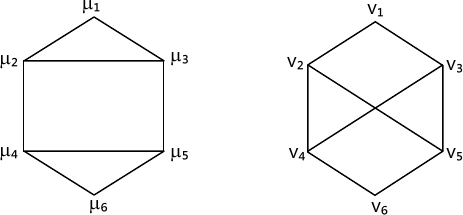ii) Find the Hamiltonian circuit using nearest neighbor method: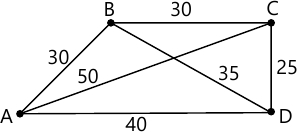6 M

4 (a) Find minimum spanning tree and its minimum weight using Prim's algorithm.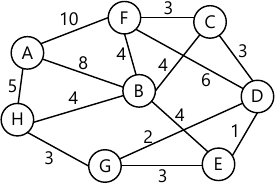6 M
4 (b) Find the shortest path using Dijkstra's algorithm for the given graphs.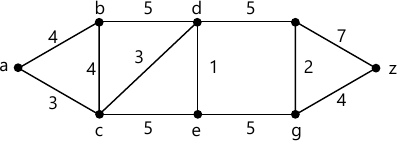6 M

Answer any one question from Q5 and Q6
5 (a) Construct an optimal binary tree for the set of weights as {15, 22, 9, 11, 10, 13, 8}. Find the weight of an optimal tree. Also assign the prefix codes and write the code words.
6 M
5 (b) Find the minimum spanning tree and weight of it for the given graph using Krushal's algorithm.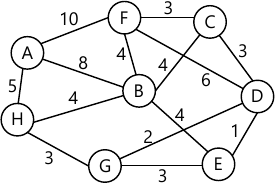7 M

6 (a) Find the maximum flow for the following transport network.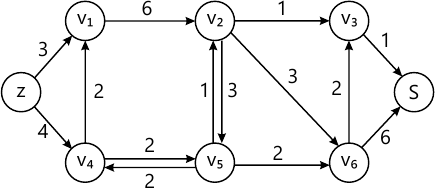6 M
6 (b) Construct a binary tree with the inorder traversal as 3, 5, 6, 7, 10, 12, 13, 15, 16, 18, 20, 23 and preorder traversal as 15, 5, 3, 12, 10, 6, 7, 13, 16, 20, 18, 23.
7 M

Answer any one question from Q7 and Q8
7 (a) A die is rolled and a coin is tossed, find the probability that the die shows an odd number and the coin shows a head.
4 M
7 (b) In how many ways can 6 men and 5 women be seated in a line so that no two women sit together?
3 M
7 (c) What is the number of ways of choosing 4 cards from a pack of 52 playing cards ? In how many of these:
(i) Four cards are of the same suit
(ii) Four cards belong to four different suits
(iii) Cards are of same colour.
6 M

8 (a) A basket contains 30 apples, 20 pears and 10 peaches. What is the probability that the first piece of fruit taken from the basket will be a peach?
4 M
8 (b) In how many ways can three prizes be distributed among four winners so that no one gets more than one prize?
3 M
8 (c) An 8 member team is to be formed from a group of 10 men and 15 women. In how many ways can the team be chosen if:
i) The team must contain 4 men and 4 women
(ii) There must be more men than women
(iii) There must be at least two men.
6 M

More question papers from Discrete Structures